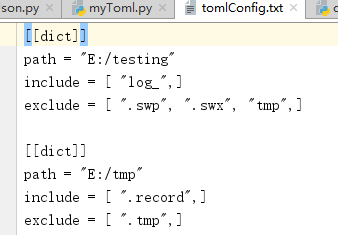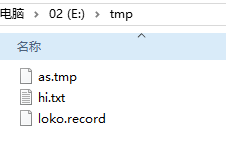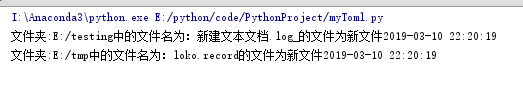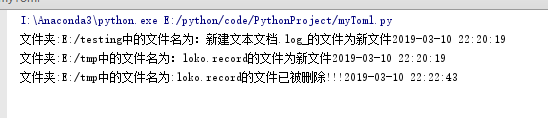# Python写一个基于MD5的文件监听程序

更新时间：2019年03月11日 10:14:59   作者：木偶Roy我要评论

MD5算法可以直接利用第三方的 hashlib 库来实现

``` m = hashlib.md5()
myFile = open(full_path, 'rb')
for line in myFile.readlines(): #以行为单位不断更新哈希值，避免文件过大导致一次产生大量开销
m.update(line) #最后可以得到整个文件的哈希值```

`class myListener(threading.Thread):`
` thread1 = myListener(mydir, json_list_include, json_list_exclude) #生成线程`

```#!/usr/bin/env python
# -*- coding:utf-8 -*-
# Author: JYRoooy
import collections
import json
import toml
if __name__ == '__main__':
myOrderDict = collections.OrderedDict
myOrderDict = {'dict':[{'path':'E:/testing', 'include':['log_'], 'exclude': ['.swp', '.swx', 'tmp']},{'path':'E:/tmp', 'include':['.record'], 'exclude': ['.tmp']}]}
myToml = toml.dump(myOrderDict, open('E:/python/code/PythonProject/tomlConfig.txt','w+'))```

toml文件```#!/usr/bin/env python
# -*- coding:utf-8 -*-
# Author: JYRoooy
import toml
import hashlib
import os
import sys
import time
import importlib

'''
监听类
'''
def __init__(self, input_dir, filt_in, filt_ex): #文件夹路径，必须包含的字符，必须过滤的字符
self.input_dir = input_dir
self.filt_in = filt_in
self.filt_ex = filt_ex
self.dict = {} #用来存储文件名和对应的哈希值
self.file_list = [] #存储每一次扫描时的文件的文件名
self.pop_list = [] #存储需要删除的文件名

def run(self):
while (1): #保证文件夹一直处于被监听的状态
for cur_dir, dirs, files in os.walk(self.input_dir):
if files != []:
self.file_list = []
for each_file_1 in files:
each_file = each_file_1
if self.filt_in: #判断文件名中是否有必须存在的字段
flagone = 0
for i in range(len(self.filt_in)):
if self.filt_in[i] in each_file:
flagone += 1
if flagone == 0:
continue

if self.filt_ex: #判断文件名中是否有必须过滤掉的字段
flagtwo = 0
for i in range(len(self.filt_ex)):
if self.filt_ex[i] in each_file:
flagtwo = 1
if flagtwo==1:
continue

self.file_list.append(each_file)
full_path = os.path.join(cur_dir, each_file)
m = hashlib.md5() #实例化md5算法

myFile = open(full_path, 'rb')

for line in myFile.readlines():
m.update(line)
if each_file not in self.dict.keys(): #如果当前的dict中没有这个文件，那么就添加进去
self.dict[each_file] = m.hexdigest() #生成哈希值
print('文件夹:' +cur_dir+ "中的文件名为：" + each_file + "的文件为新文件" + time.strftime('%Y-%m-%d %H:%M:%S',
time.localtime(time.time())))
if each_file in self.dict.keys() and self.dict[each_file] != m.hexdigest(): #如果当前dict中有这个文件，但是哈希值不同，说明文件被修改过，则需要对字典进行更新
print('文件夹:' +cur_dir+ "中的文件名为：" + each_file + "的文件被修改于" + time.strftime('%Y-%m-%d %H:%M:%S',
time.localtime(time.time())))
self.dict[each_file] = m.hexdigest()
myFile.close()
pop_list = []
for i in self.dict.keys():
if i not in self.file_list: #当字典中有不在当前文件名列表中时，说明文件已经被删除
print('文件夹:' +cur_dir+ '中的文件名为:' + i + "的文件已被删除!!!" + time.strftime('%Y-%m-%d %H:%M:%S',
time.localtime(time.time())))
pop_list.append(i)
for i in pop_list:
self.dict.pop(i)

time.sleep(2)

if __name__ == '__main__':
threads = [] #用来存储线程的线程池
with open('E:/python/code/PythonProject/tomlConfig.txt','r+') as f: #读取toml格式的文件，并分解格式
myList = mytoml['dict']
for i in range(len(myList)): #因为可能同时需要监听多个文件夹，所以利用线程池处理多线程
json_list_include = []
json_list_exclude = []
mydir = myList[i]['path']
for sublist in range(len(myList[i]['include'])):
json_list_include.append(myList[i]['include'][sublist])
for sublist in range(len(myList[i]['exclude'])):
json_list_exclude.append(myList[i]['exclude'][sublist])
thread1 = myListener(mydir, json_list_include, json_list_exclude) #生成线程

for t in threads: #开启所有线程
t.start();```